# Denotational semantics

You’re given diagrams of some Haskell types ordered by semantic order. Vertices are inhabitants of a datatype, and the lines point upwards, from less defined to more defined. (See Wikibooks for an introduction and examples.) Your objective is to construct a type corresponding to the diagram, or show that is impossible.

I’ve done the first one – it is obviously Bool. Good luck!

 Diagram Type`Bool`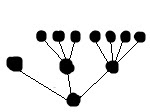Solution `data Three = X | Y | Zdata Four = A | B | C | Ddata T = P | Q Three | R Four` Using this method you can define any finite tree.Solution `data NaturalsUp = S NaturalsUp`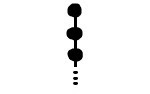Solution Impossible. There is no bottom element.Solution `type NaturalsDown = NaturalsUp -> ()`Solution `data Wrap = Wrap ktype T = Wrap (Wrap (Maybe NaturalsUp)`Solution `Integer`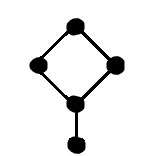Solution `((),())`Solution `data T = L T | R T`Solution Impossible. The type must form a complete partial order – you could carry the computation indefinitely long, and get the infinite path.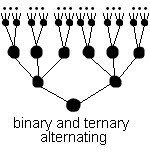Solution `data T1 = A T2 | B T2data T2 = C T1 | D T1 | E T1`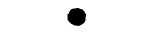Solution `data Void` An empty data type has no inhabitants other than bottom. It is used in phantom types.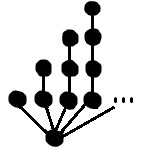Solution `data Voiddata B k = B k deriving (Show)data T x = P x | A !(T (B x)) deriving (Show)type X = T Void` The tree looks like:The type system is quite expressive – for example, the depths could form only perfect squares.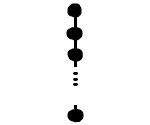This is a variant of NaturalsDown and will require cheating a little. Create a module that will export a data type, and only a “smart constructor” that will equate undefined and const undefined. `module M (Fun, Nat(..), create) wheredata Nat = Zero | S Natnewtype Fun = Fun (Nat -> ())infinity = S infinitycreate :: (Nat -> ()) -> Funcreate f = seq (f infinity) (Fun f)` Outside the module, you cannot create Fun (const undefined).

Application

A great application of denotational semantics is doing numerical integration exactly. Continua a leggere

Pubblicato in Senza categoria

# Abstract Types vs Parameter

This topic (and the next) are intended to discuss abstract types. A class/trait with an abstract type is quite similar to a class/trait type parameter. For example:trait C[A] {  def get : A  def doit(… Continua a leggere

Pubblicato in Senza categoria